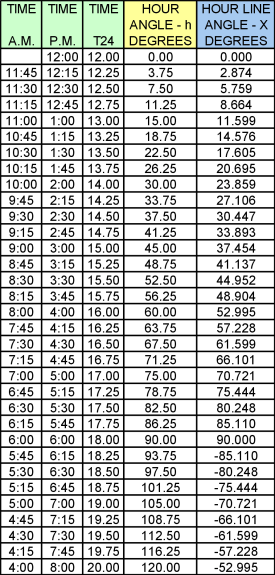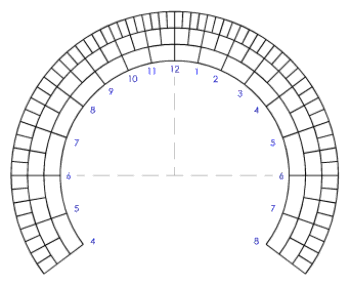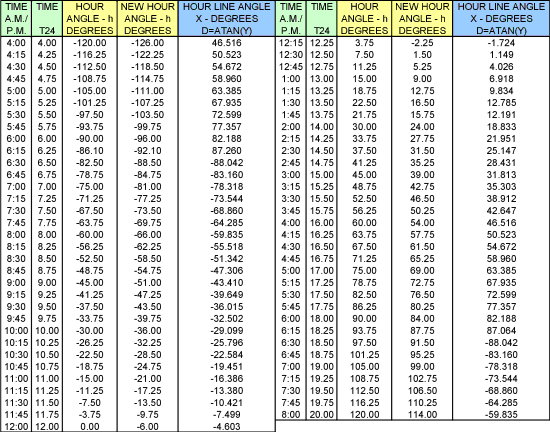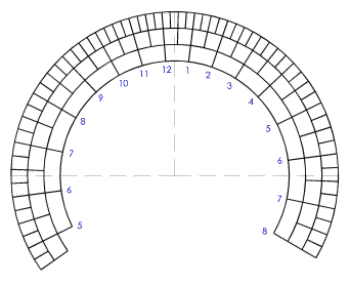The Sundial Primer created by Carl SabanskiThe Sundial Primer Index
 Longitude Correction Longitude Correction: is the correction required to local apparent time (L.A.T.) to translate it to the L.A.T. for the central meridian for that time zone.Standard Time Zone: a geographical region which uses the same civil (clock) time. These are approximately regions between two lines of longitude, set 15° apart, and hence 1 hour time between adjacent zones. The standard time is the mean solar time at the central or standard meridian for the zone. For the UK, which is in Zone 0, the standard meridian is the Prime Meridian at Greenwich, and the zone extends nominally 7½° west to 7½° east.Mean Solar Time: is a measure of time base conceptually on the diurnal motion of the fictitious mean Sun, under the assumption that the Earth's rate of rotation is constant.Local Apparent Time (L.A.T.): is solar time, as derived from the real Sun at any particular location.Zonal Solar Time: is the solar time at a time zone meridian. It is local apparent time (L.A.T.) with a longitude correction but without the Equation of Time. This discussion will deal with a horizontal sundial but applies to other sundials where longitude correction can be applied. The hour line angles (X, HLA) for a horizontal sundial can be calculated as follows: X = arctan {sin ø * tan (h)} where h is the hour angle, in degrees, given by:h = (T24 - 12) * 15°and T24 is the time in 24-hour clock notation (hours after midnight) in decimal hours.Table 1 illustrates this calculation for a dial located at 50°N latitude. No corrections have been applied, so this dial will indicate local apparent time.Table 1: Hour Line Angle CalculationsFigure 1 is a template of the hour lines. This can be used to construct the sundial. Notice that the A.M. and P.M. hour lines are symmetrical about the noon hour line.Figure 1: Horizontal Sundial - No Corrections (ZW2000/CAD) A correction for longitude is made by adjusting the hour angle (h) prior to calculating the hour line angles (X). This will result in moving the hour lines counter-clockwise if the dial is located west of the standard meridian for the time zone and clockwise if it is located east. The correction is calculated as follows:Longitude Correction = (Standard Meridian - Local Meridian)°This value is negative for a location west of the standard meridian and positive for an east location. This value is added to all the hour angles to obtain the new corrected hour angles.Table 2 illustrates this correction for a dial located at 50°N latitude and 96°W longitude. The standard meridian for the time zone is 90°W. The correction to be added is: Longitude Correction = (90 - 96) = -6°Table 2: Hour Line Angle Calculations - Longitude Correction As each time zone is 15° apart and there is a 1 hour time difference between adjacent zones, then every 1° is equivalent to 4 minutes of time. In this example, the longitude correction of -6° means that the hour lines are shifted 24 minutes earlier. Figure 2 illustrates this shift. This template can be used to construct a dial that has been corrected for longitude and indicates zonal solar time.Figure 2: Horizontal Sundial - Longitude Correction (ZW2000/CAD)You can download a spreadsheet that will perform these calculations for you. You can find these, where available, on the page dealing with a particular type of sundial.